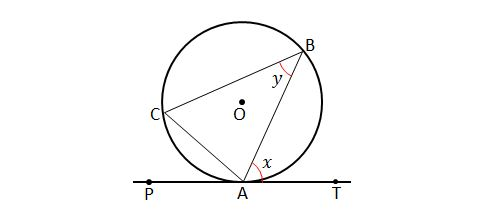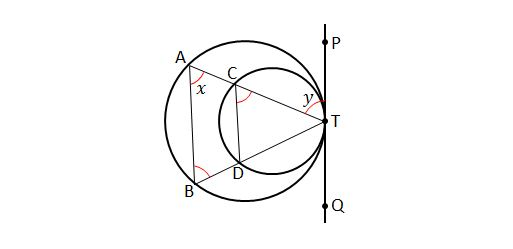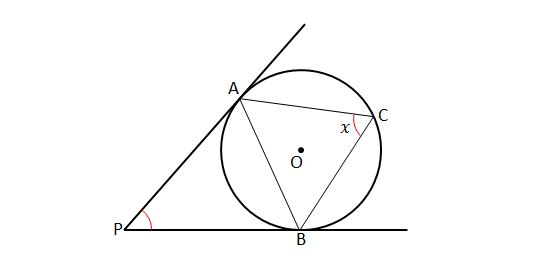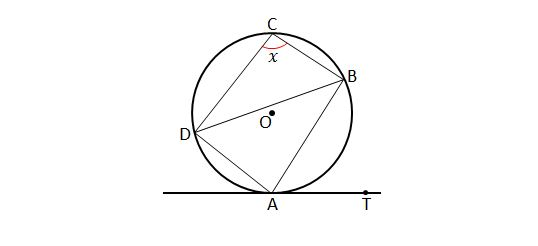Geometry

# Alternate Segment TheoremIn the above diagram, $\overline{ PAT}$ is tangent to circle $O$ at point $A.$ If $\angle BCA={56}^\circ \text{ and } \angle CAP={49}^\circ,$ what are the measures of the angles $\angle x$ and $\angle y ?$

Note: The above diagram is not drawn to scale.If $\overline{AT}$ is tangent to circle $O$ with radius $4$ at $A$ and $\angle BAT=60^\circ,$ what is the area of $\triangle OAB ?$In the above diagram, if $\overline{PTQ}$ is tangent to both circles at $T$ and $\angle ABT={72}^\circ, \angle DCT={76}^\circ,$ what is the value of $\angle x+\angle y$ in degrees?If $\overline{PA}$ and $\overline{PB}$ are tangent to circle $O$ at points $A$ and $B,$ respectively, and $\angle APB={68}^\circ,$ what is the measure of $\angle x$ in degrees?

Note: The above diagram is not drawn to scale.In the above diagram, quadrilateral $ABCD$ is inscribed in circle $O$ and $\overline{AT}$ is tangent to circle $O$ at point $A.$ If $\angle BAT={59}^\circ$ and $\angle DBA={39}^\circ,$ what is the measures of $\angle x$ in degrees?

Note: The above diagram is not drawn to scale.

×